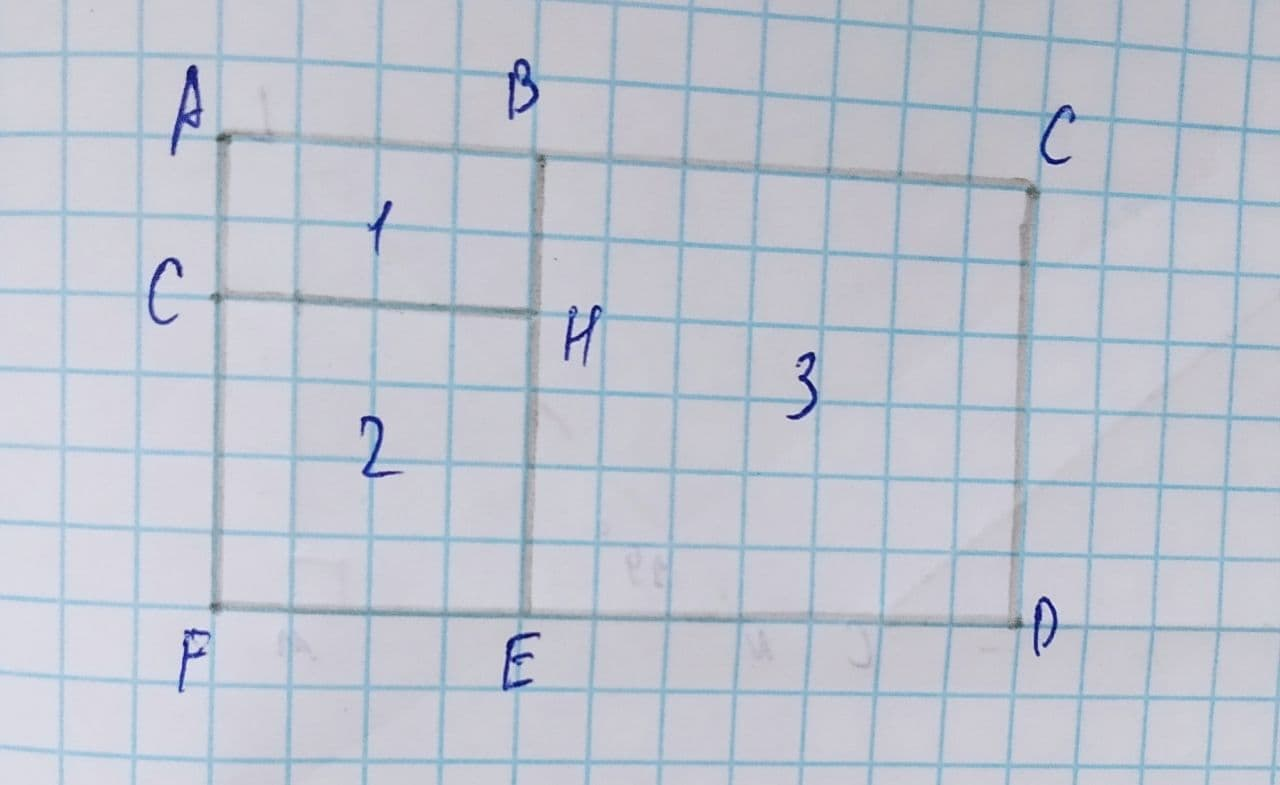Question# Determine the perimeter of rectangle ACDF.

Similarity
ANSWEREDIn the figure on the right, rectangles 1, 2 and 3 are similar.
Determine the perimeter of rectangle ACDF.
Given:
The figureAnd AG=4 cm and GF=9 cm2021-08-08
Then,
AF=AG+GF
=4+9
=13
Since AF=CD, this implies CD=13 cm,
From the figure, it is observed that rectangle 2 and 3 are square, this implies
GF=HE=9 cm
And
CD=ED=13 cm
Therefore, FD=FE+ED=9+13=22 cm and FD=AC=22 cm
Then, the perimeter of rectangle ACDF is AC+CD+DF+FA
=22+13+22+13
=70
Thus, the perimeter of rectangle ACDF is 70 cm.